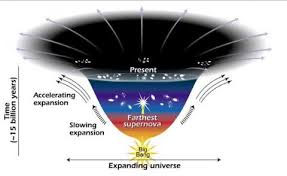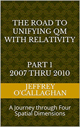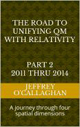# Dark Energy as a displacement in the fabric of space-time

In the early 1990s, one thing was fairly certain about the expansion of the universe. It might have enough energy density to stop its expansion and recollapse, it might have so little energy density that it would never stop expanding, but gravity was certain to slow the expansion as time went on. Granted, the slowing had not been observed, but, theoretically, the universe had to slow. The universe is full of matter and the attractive force of gravity pulls all matter together. Then in 1998 observations  made by the Hubble Space Telescope (HST) of very distant supernovae that showed that, a long time ago, the universe was actually expanding more slowly than it is today. So the expansion of the universe has not been slowing due to gravity, as everyone thought, it has been accelerating. No one expected this, no one knew how to explain it. But something was causing it.

More is unknown than is known. We know how much Dark Energy there is because we know how it affects the universe’s expansion. Other than that, it is a complete mystery. But it is an important mystery. It turns out that roughly 68% of the universe is dark energy. Dark matter makes up about 27%. The rest – everything on Earth, everything ever observed with all of our instruments, all normal matter – adds up to less than 5% of the universe. Come to think of it, maybe it shouldn’t be called "normal" matter at all, since it is such a small fraction of the universe.

According to Nasa’s universe web site one explanation for Dark Energy, as Albert Einstein suggested is that it is a property of space.  In other words he was the first person to suggest that empty space is not nothing.  The first property that Einstein discovered is that it is possible for more space to come into existence. Then one version of Einstein’s gravity theory, the version that contains a cosmological constant, makes a second prediction: "empty space" can possess its own energy. Because this energy is a property of space itself, it would not be diluted as space expands. As more space comes into existence, more of this energy-of-space would appear. As a result, this form of energy would cause the universe to expand faster and faster. Unfortunately, no one understands why the cosmological constant should even be there, much less why it would have exactly the right value to cause the observed acceleration of the universe"

However following Einstein lead we may find the answer these questions by attempting to understand exactly how and why space is expanding in terms of his space-time model.

For example Einstein’s Special and General Theories of Relativity is based on the relative simple concept of a space-time metric. Granted the math required to determine the how space and time interact on objects can be very complicated however understanding or visualizing how space-time effect them is relative easy to do.

However one of the difficulties in understanding how three-dimensional properties of space are expanding is defining what it is expanding into because only place it can go according his theories is towards a time dimension.

Granted one can assume that space is expanding relative to itself but that would mean that it must extend to infinity because if it did have a boundary its outer most region would have to be moving with respect to something.

However, the theoretical model called the Big Bang which most cosmologists accept as the definitive origin for both space and time tell us that it cannot be infinite because it assumes its expansion began not from and infinite volume but form one with boundaries called a singularity.

Additionally because three-dimensional space is undergoing a spatial expansion it is difficult to understand how three-dimensional space can physically expanding towards time dimension because time does not have physical physical properties of the spatial dimensions.

But Einstein gave us the ability to develop a better understanding of the expansion of three dimensional space when he used the equation E=mc^2 and the constant velocity of light to define the geometric properties of mass in a space-time universe because that provided a method of converting a unit of time he associated with energy in space-time to unit of space one can associate with mass in four *spatial* dimensions.  Additionally because the velocity of light is constant he also defined a one to one quantitative correspondence between his space-time universe and one made up of four *spatial* dimensions.

This fact that one can use Einstein’s theories to qualitatively and quantitatively derive the *spatial* properties of time in space-time universe in terms of four *spatial* dimensions is one the bases of assuming as was done in the article “Defining energy” Nov 27, 2007 that all forms of energy can be derived in terms of a *spatial* displacement in a "surface" of a three-dimensional space manifold with respect to a fourth *spatial* dimension.

In other words one can not only use Einstein’s equations to quantitatively and qualitatively derive how energy interacts with time in a space-time dimension but also how it would interact with its *spatial* equivalent in four *spatial* dimensions.

In other words his theories tell us that three-dimensional space can expand towards a fourth *spatial* dimension as well as a time dimension.

We know from the study of thermodynamics that energy flows from areas of high to ones with low density very similar to how water flows form an elevated or "high density" point to a lower one.

For example if the walls of an above ground pool filled with water collapse the elevated two-dimensional surface of the water will flow or expand and accelerate outward towards the three-dimensional environment sounding it.

Yet we know from observations of the cosmic background radiation that presently our three-dimensional universe has an average energy component equal to about 3.7 degrees Kelvin.

However this means that according to concepts developed in the article “Defining energy" (mentioned earlier) the three-dimensional "surface" of our universe which has an average energy component of 3.7 degree Kelvin would be elevated with respect to a fourth *spatial* dimension.

Similar to the water in a pool whose sides had collapsed, if the "surface" of a three-dimensional manifold was displaced with respect to a fourth *spatial* dimension as Einstein tell us as it must be if one redefines his space-time universe in terms of four *spatial* dimension then it would be accelerated outward.

In other words one qualitatively understand the casually of the accelerated expansion of our universe in term of water accelerating out of collapsed pool.

This suggests the accelerative force dark energy is caused by the fact that the base line Einstein used to define energy in a space time is displaced with respect to its zero point.

Some many question this because if true it means that over time the expansion rate should decrease because similar to the water in a pool its velocity should decrease as it expands and its energy dissipates.

However the universe’s expansion to appears to be accelerating. as the graphic shows, instead of slowing down as this model suggests it should.  The reason is because it assumes there are two factors that determine its expansion rate: the attractive forces of gravity which would cause it to slow and Dark Energy which case it to expand.However since gravitational forces are inversely proportional to distance its effects are reduce by the increasing distance between their components cause by the physical expansion of space and the kinetic energy imparted to its gravitational mass components by the big bang while if the above model is correct the only thing that effects Dark Energy is the magnitude of the *spatial* displacement of three-dimensional space with respect to fourth *spatial* dimensions. In other words the contractive forces of gravity should be according the above model decreasing faster that the expansive forces of Dark Energy because of their increase rate of separation relative to dark energy Therefore the expansive force of dark energy would appear to be increasing even though it may be constant or decreasing over the course of time.

This could be verified by determining the ratio of the energy is lost by the gravitational force as a result of the universe’s expansion and kinetic energy imparted to the its mass components and see if it corresponds to the energy required to explain the observed increase in its acceleration.

In other words one can explain the observed properties of Dark Energy in terms of a *spatial* displacement a "surface" of three dimensional space with respect to a fourth *spatial* dimension as well as with respect to a time dimension because in Einstein’s mathematics tells us they are interchangeable.

Some may also feel that this is an over simplification of what appears on the surface to be a rather complex phenomena such as Dark Energy but is no more simplistic that the one use to help us understand how gravity works in a space-time environment. Granted the math behind this concept may be complex and difficult to understand as it is with the gravitational curvature in space-time however that does not mean that we cannot use it to understand its causality.

It should be remember that Einstein’s genius and the symmetry of his mathematics allows us to choose whether to define the forces associated with gravity and dark energy in either four *spatial* dimensions or four dimensional space-time.

Later Jeff

 The Road to Unifying QM with Relativity part 1 2007 thru 2010Ebook \$8.00Paper Back \$15.00 The Road to Unifying QM with Relativity part 2 2011 thru 2014Ebook \$8.00Paper Back \$16.00 The Road to Unifying QM with Relativity part 3 2015 thru 2020Ebook \$8.00Paper Back \$18.00